# An Example of matrixset in Action

We will use as an example a data set taken from Aiyetan et al., a synthetic MRM targeted proteomic data set.

The goal here is to showcase the matrixset capacities and not to delve into what is MRM or how to analyze such a data set. The reference paper and its bibliography is actually a good place to learn more about this technology.

We will also not try to replicate the methodologies developed in the paper.

For the purpose of this showcase, here is a very oversimplified description of MRM:

• A set of analytes - called transitions - is measured for each sample. The analytes are combinations of peptides/ions and thus a natural grouping of the analytes (the peptides) exists.

• Each analyte has a companion analyte measured as well, called the heavy transition1. Its purpose is to serve as a normalizer for the transition, which we refer to, by opposition, as the light transition.

Note that while the ratio of light over heavy measures is a more stable measure in many aspects, further normalization is usually required.

The samples of this data set consist of seven dilution ranges performed in triplicates, in an assay of 15 peptides, each with 3 transitions, for a total of 45 analytes.

Here is a view of the data as it exists in matrixset:

mrm_plus2015
#> matrixset of 4 30 × 45 matrices
#>
#> matrix_set: light_area
#> A 30 × 45 <dbl> matrix
#> Blank_0_1              59322             86331 ...         142065456
#>       ...                ...               ... ...               ...
#> Blank_3_2              29640             46116 ...         103866312
#>
#> matrix_set: heavy_area
#> A 30 × 45 <dbl> matrix
#> Blank_0_1                  0                 0 ...                 0
#>       ...                ...               ... ...               ...
#> Blank_3_2                  0                 0 ...             43674
#> And 2 other matrices named "light_rt" and "heavy_rt"
#>
#> row_info:
#> # A tibble: 30 × 5
#>    RunOrder .rowname  SampleType     Replicate   CalibrationPoint
#>       <int> <chr>     <chr>          <chr>       <chr>
#>  1        1 Blank_0_1 Blank          <NA>        <NA>
#>  2        2 Blank_0_2 Blank          <NA>        <NA>
#>  3        3 Blank_0_3 Blank          <NA>        <NA>
#>  4        4 A_1       SerialDilution Replicate_1 Point_1
#>  5        5 B_1       SerialDilution Replicate_1 Point_2
#>  6        6 C_1       SerialDilution Replicate_1 Point_3
#>  7        7 D_1       SerialDilution Replicate_1 Point_4
#>  8        8 E_1       SerialDilution Replicate_1 Point_5
#>  9        9 F_1       SerialDilution Replicate_1 Point_6
#> 10       10 G_1       SerialDilution Replicate_1 Point_7
#> # ℹ 20 more rows
#>
#>
#> column_info:
#> # A tibble: 45 × 9
#>    PeptideSequence PrecursorCharge ProductCharge FragmentIon light PrecursorMz
#>    <chr>                     <dbl>         <dbl> <chr>       <chr>
#>  1 AGPNGTLFVADAYK                2             1 y10         712.856449
#>  2 AGPNGTLFVADAYK                2             1 y8          712.856449
#>  3 AGPNGTLFVADAYK                2             1 y7          712.856449
#>  4 DIENFNSTQK                    2             1 y8          598.775125
#>  5 DIENFNSTQK                    2             1 y7          598.775125
#>  6 DIENFNSTQK                    2             1 y6          598.775125
#>  7 FNETTEK                       2             1 y6          435.19799
#>  8 FNETTEK                       2             1 y5          435.19799
#>  9 FNETTEK                       2             1 y4          435.19799
#> 10 YLGNATAIFFLPDE…               2             1 y8          878.943253
#> # ℹ 35 more rows
#> # ℹ 4 more variables: light ProductMz <chr>, heavy PrecursorMz <chr>,
#> #   heavy ProductMz <chr>, .colname <chr>

The first thing we will do is to create a new measure that is the ratio, on the log scale, of the light and heavy transitions.

As seen below, this is an easy operation with matrixset. Note that we take the opportunity to map the calibration points with their dilution values and assign point/replicate ID to the blanks.

library(tidyverse)
mrm_plus2015_ratio <- mrm_plus2015 %>%
mutate_matrix(area_log_ratio = log(light_area+1) - log(heavy_area+1)) %>%
annotate_row(dilution = case_when(CalibrationPoint == "Point_1" ~ 57.6,
CalibrationPoint == "Point_2" ~ 288,
CalibrationPoint == "Point_3" ~ 1440,
CalibrationPoint == "Point_4" ~ 7200,
CalibrationPoint == "Point_5" ~ 36000,
CalibrationPoint == "Point_6" ~ 180000,
CalibrationPoint == "Point_7" ~ 900000,
TRUE ~ NA_real_)) %>%
annotate_row(point = case_when(is.na(CalibrationPoint) ~ str_match(.rowname, "(.*)_\\d$")[,2], TRUE ~ CalibrationPoint), replicate = case_when(is.na(CalibrationPoint) ~ paste("Replicate", str_match(.rowname, ".*_(\\d)$")[,2], sep = "_"),
TRUE ~ Replicate))

This is a linearity experiment, so the first thing we can try is to look at the linearity of the first analyte (arbitrary choice).

mrm_plus2015_ratio[,1,] %>%
filter_row(!is.na(CalibrationPoint)) %>% # discards the blanks
apply_matrix(~ {
d <- current_row_info()
d$AGPNGTLFVADAYK|y10 <- .m[,1] ggplot(d, aes(log(dilution), AGPNGTLFVADAYK|y10)) + geom_point() }, .matrix = "area_log_ratio") #>$area_log_ratio
#> $area_log_ratio$~...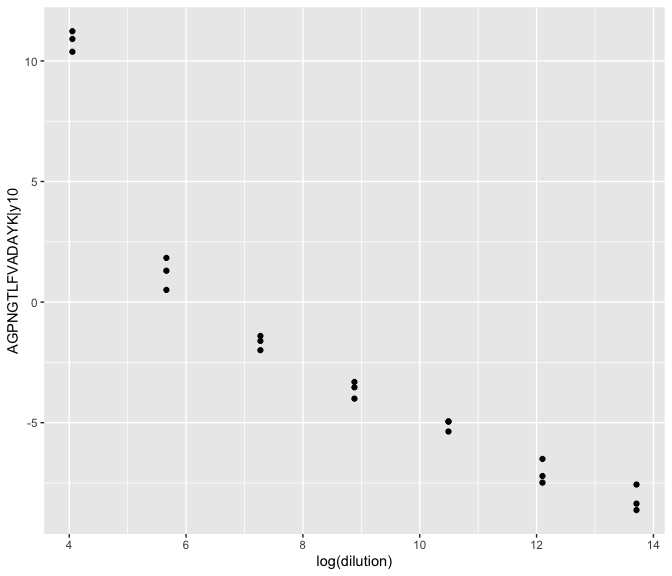We could also look at it more globally. For instance, we could average the triplicates and look at all the analytes at once, applying a global smoother.

This is a bit more challenging as we need to put together the matrix averaged values and the meta information (annotation). A trick we can use is to put the average results in the annotation frame with annotate_column_from_apply.

mrm_plus2015_ratio %>%
# to average the triplicates
row_group_by(CalibrationPoint) %>%
# send the analyte averaged values in the annotation frame
annotate_column_from_apply(foo = mean(.j), .matrix = "area_log_ratio") %>%
column_info() %>%
select(-NA) %>% # discards the blanks
pivot_longer(Point_1:Point_7, names_to = "CalibrationPoint", values_to = "Rep_avr") %>%
left_join(mrm_plus2015_ratio %>%
row_info() %>%
select(-.rowname, -Replicate, -RunOrder) %>%
distinct()) %>%
ggplot(aes(log(dilution), Rep_avr)) +
geom_point() +
geom_smooth()
#> Joining with by = join_by(CalibrationPoint)
#> Warning in left_join(., mrm_plus2015_ratio %>% row_info() %>% select(-.rowname, : Detected an unexpected many-to-many relationship between x and y.
#> ℹ Row 1 of x matches multiple rows in y.
#> ℹ Row 4 of y matches multiple rows in x.
#> ℹ If a many-to-many relationship is expected, set relationship =
#>   "many-to-many" to silence this warning.
#> geom_smooth() using method = 'loess' and formula = 'y ~ x'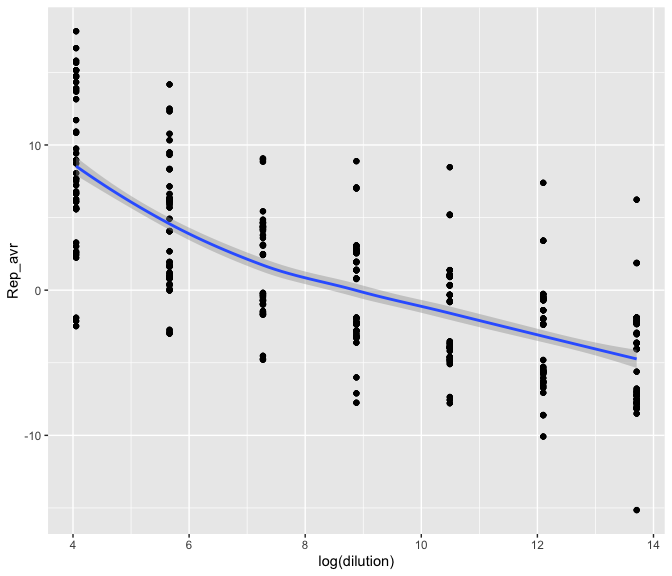An alternative when the data is not too big is to first convert the object to a data frame. This would give an identical result.

mrm_plus2015_ratio %>%
ms_to_df(.matrix = "area_log_ratio") %>%
filter(!is.na(CalibrationPoint)) %>%
group_by(dilution, .colname, CalibrationPoint) %>%
summarise(Rep_avr = mean(.vals)) %>%
ggplot(aes(log(dilution), Rep_avr)) +
geom_point() +
geom_smooth()

A classic in Exploratory Data Analysis is to perform a PCA. To do so, we can exploit the apply_matrix() function that uses the matrix input format and feed to the PCA. Note that we use default centering and scaling but that better suited pre-processing may apply and be investigated.

library(patchwork)
library(magrittr)
library(ggfortify)

pcas <- mrm_plus2015_ratio %>%
mutate_matrix(light_area = log(light_area+1),
heavy_area = log(heavy_area+1)) %>%
apply_matrix(pca = ~ {
list(meta=current_row_info(), pca=prcomp(.m, scale. = TRUE))
}, .matrix = c("light_area", "heavy_area", "area_log_ratio"))

bps <- imap(pcas, ~ autoplot(.x$pca$pca, data = .x$pca$meta,
colour = "point") +
ggtitle(.y))

(bps[] / bps[] / bps[]) + plot_layout(guides = "collect")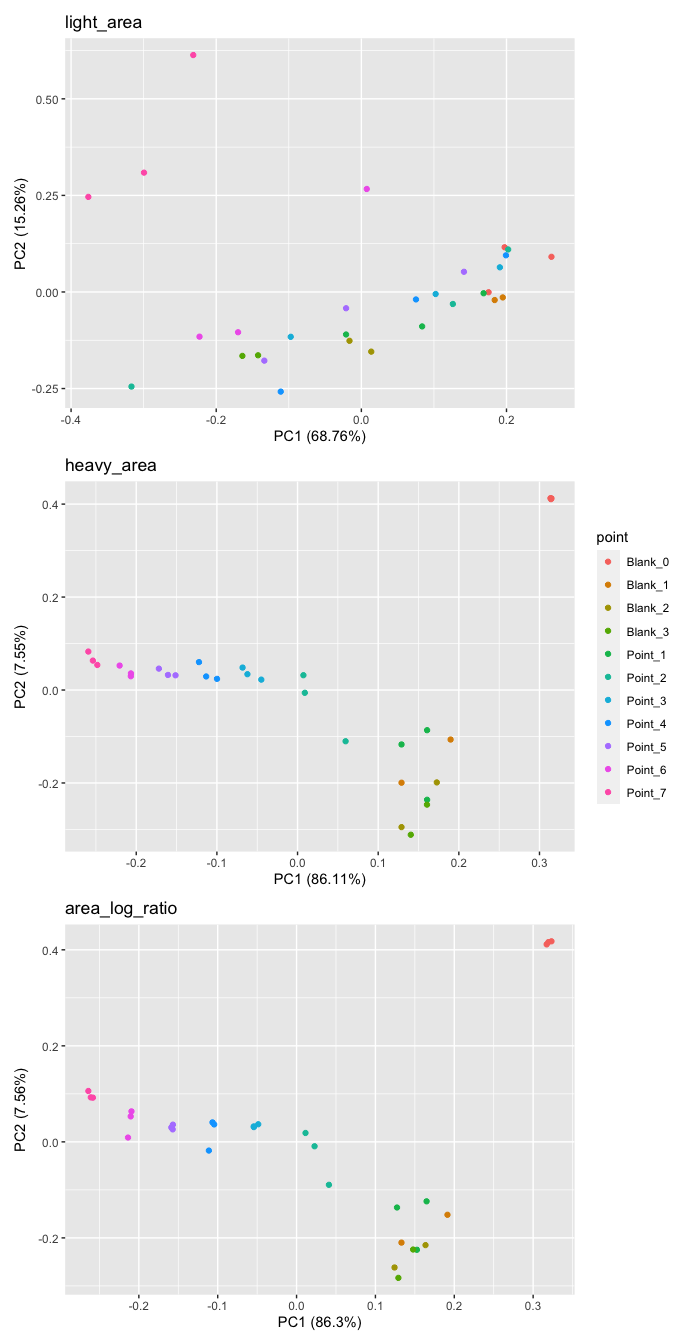We can easily see the triplicate nature of the data. We can also how separable are the calibration points.

We can also see that a blank sample behaves differently than the other samples.

Part of the explanation may be found in the fact that these blank samples are less reliably measured than the other samples.

To make this assessment, we tallied the fraction of analytes among samples with a measure of at least 50000 (more or less arbitrary threshold). The result is shown below.

mrm_plus2015 %>%
apply_row_dfl(poor = sum(.i < 50000),
.matrix = c("light_area", "heavy_area")) %>%
bind_rows(.id = "area") %>%
ggplot(aes(.rowname, poor, fill = area)) +
geom_col(position = "dodge") +
labs(y = "% < 50000") +
theme(axis.text.x = element_text(angle = 90))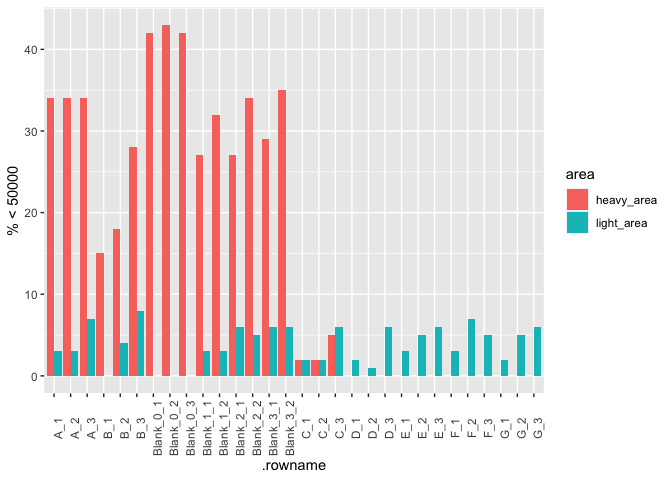Another thing we can look at is the sample distribution. While many visual methods can be used for that purpose, boxplots are among the simplest.

A challenge here is that for the geom_boxplot() function to draw a box per sample, we would need the annotation and values to be in the same data frame.

A trick is to pre-compute the boxplot stats and provide them to geom_boxplot(). We take the opportunity to showcase the possibility of using non-standard evaluations. The advantage is that later in this vignette, we will re-use the expressions.

box_expr <- list(ymin = expr(min(.i)),
lower = expr(quantile(.i, probs = .25, names = FALSE)),
middle = expr(median(.i)),
upper = expr(quantile(.i, probs = .75, names = FALSE)),
ymax = expr(max(.i)))

mrm_plus2015_ratio %>%
annotate_row_from_apply(.matrix = area_log_ratio,
!!!box_expr) %>%
row_info() %>%
ggplot(aes(.rowname,
ymin = ymin,
lower = lower,
middle = middle,
upper = upper,
ymax = ymax)) +
geom_boxplot(stat = "identity") +
theme(axis.text.x = element_text(angle = 90))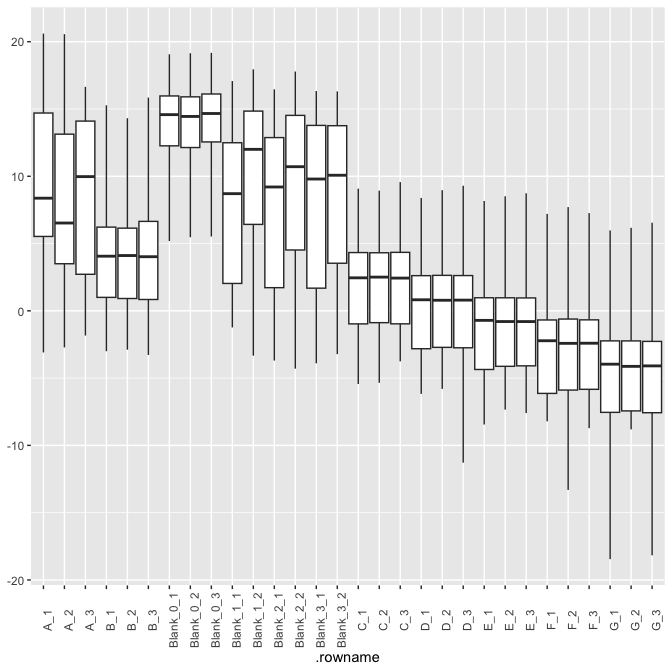Just for fun, let us now pretend that it is a good idea to normalize this data set to remove the dilution effect.

We will proceed by fitting a linear mixed model with a fixed effect for sample type (blank or not) and random batch effect for calibration point.

library(lme4)
diff <- mrm_plus2015_ratio %>%
apply_column_dfl(p_cov = predict(lmer(.j ~ 1 + SampleType + (1|point)),
type = "response"),
p_null = predict(lm(.j ~ 1)),
.matrix = "area_log_ratio") %>%
.[] %>%
mutate(d = p_cov - p_null) %>%
select(.colname, .rowname = p_cov.name, d) %>%
pivot_wider(names_from = ".colname", values_from = "d") %>%
column_to_rownames(".rowname") %>%
data.matrix()

mrm_plus2015_ratio %<>%
mutate_matrix(norm_log_ratio = area_log_ratio - diff) 

We can now look at the impact of our procedure.

mrm_plus2015_ratio %>%
annotate_row_from_apply(.matrix = norm_log_ratio,
!!!box_expr) %>%
row_info() %>%
ggplot(aes(.rowname,
ymin = ymin,
lower = lower,
middle = middle,
upper = upper,
ymax = ymax)) +
geom_boxplot(stat = "identity")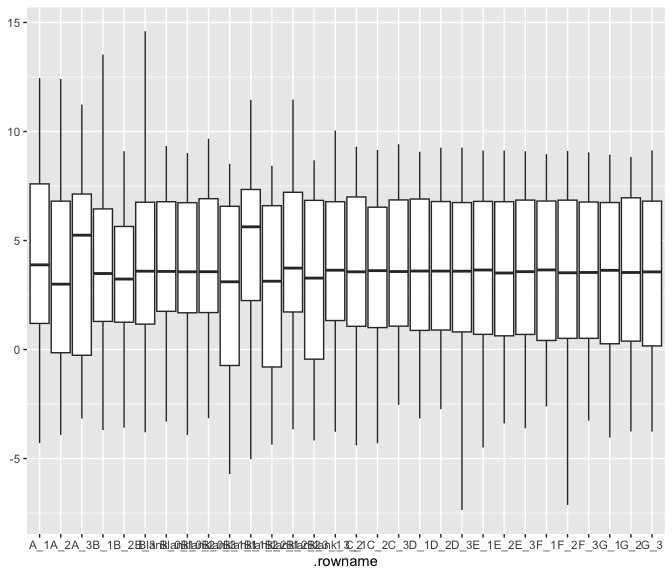pca <- mrm_plus2015_ratio %>%
apply_matrix(pca = ~ {
list(meta=current_row_info(), pca=prcomp(.m, scale. = TRUE))},
.matrix = "norm_log_ratio") %>%
.[] %>%
.$pca autoplot(pca$pca, data = pca\$meta, colour = "point")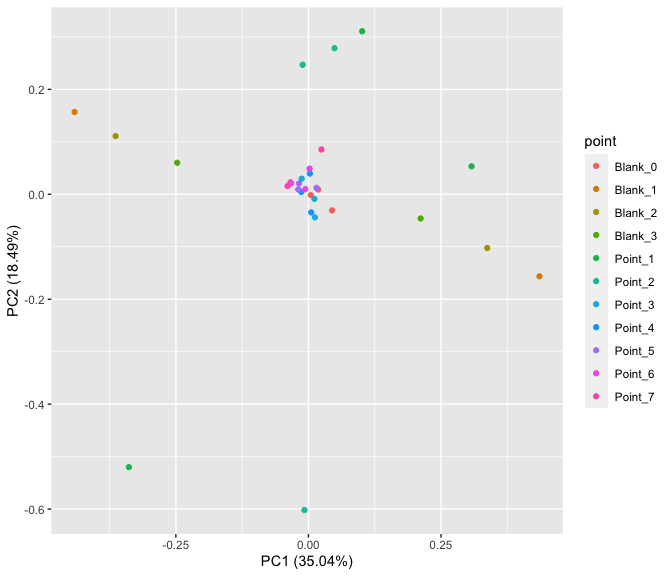1. If you are interested in knowning more on the topic, search for MRM and light and heavy-labeled peptides.↩︎